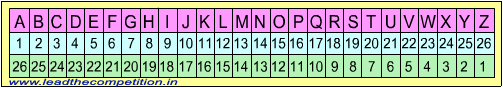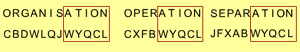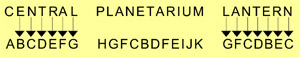#### Test of Reasoning - Questions on Codes1. If GOLD is written as IQNF, how WIND can be written in the code?

Explanation:Answer (a). The code followed is A --> C; B --> D; .... G --> I; W --> Y etc. Shortcut: D is common in both GOLD and WIND, and F is common at the last position in both IQNF and YKPF.

2. If HKUJ means FISH, what does UVCD mean?

Explanation:Answer (b). The code followed is H --> F; K --> I; U --> S; J --> H (i.e. second letter backwards). Thus U --> S; V --> T; C --> A; D --> B.

3. If NOIDA is written as STNIF, how can MEERUT be written in that code?

Explanation:Answer (c). Each letter is replaced by the 5th letter following it. Thus N is replaced by S leaving O, P, Q and R in between.

4. In a certain code FORGET is written as DPPHCU, how would DOCTOR be written in that code?

Explanation:Answer (a). The letters at odd places i.e. 1st, 3rd, 5th are moved backwards by two letters. Thus F becomes D; R becomes P and E becomes C and the letter at even place moves one letter forward, thus O becomes P, F becomes H and T becomes U. Therefore in DOCTOR, D would become B, C would become A and O would become M. Only option (d) satisfies the condition.

5. In a certain code DECEMBER is written as ERMBCEDE, in that code which word will be written as ERMBVENO?

6. If RED is coded as 6720, then how GREEN would be coded?

RED -> DER -> 4 - 5 - 18 -> 6 - 7 - 20
GREEN -> NEERG -> 14 - 5 - 5 - 18 - 7 -> 16 - 7 - 7 - 20 - 9.

7. If ROSE is written as TQUG, how BISCUIT can be written in that code?

Explanation:Answer (d). Each letter is replaced by the second letter following it. R is replaced by T and B is replaced by D.

8. If the word MENTAL is written as LNDFMOSUZBKM, then how would the word TEST be written in that code?

Explanation:Answer (d). Each letter is replaced by two letters - one preceding and the other succeeding it. Thus for T, S and U are used and for E, D and F are used.

9. If TYPEWRITER is written as GBKVDIRGVI, how STENO can be written in that code?

Explanation:Answer (b). The letters are replaced with letters in the same position from the end. Thus T which is 20th letter reading from A is replaced by G which is the 20th letter reading from Z. See the image on the top of the page.

10. If KASHMIR is written as 8142753, how can RIMSHAK be written in that code?

Explanation:Answer (a). We need to just replace the words with numbers in proper order. R represents number 3 simillarly I -> 5, M -> 7, S -> 4, H -> 2 and K -> 8. Hence the answer is 3-5-7-4-2-1-8

11. If in a code language as ORGANISATION is written as CBDWLQJWYQCL and OPERATION is written as CXFBWYQCL, how is SEPARATION coded?

Explanation:Answer (c). Without going into detailed decoding, it can be seen that only answer (c) has the common alphabets WYQCL which correspond to common leters TION in the given words.12. In a particular way of coding, the word CENTRAL is coded as ABCDEFG and PLAN-ETARIUM as HGFC-BDFEIJK. With the same coding how can we express the word LANTERN?

Explanation:Answer (b).From the code given for CENTRAL, it is clear that separate alphabets have been assigned to each alphabet in the original word. It is also seen that the word LANTERN can be formed from the word central.13. In a certain code language BLOOD is written as EIRLG. How will the word PERIOD be written in that code language?

Explanation:Answer (b). Letters in the odd place (B, O and D) are replaced with a letter 3 places forward in the alphabet and letters in the even place (L and O) are replaced with a letter 3 places behind in the alphabet. Thus P (in the word PERIOD) is written as S (leaving Q and R) and D is written as A (leaving C and B). That leaves us with only on choice i.e. (b).

14. In a certain code language INCORPORATE is written as HCGJSLJSXHO and PELMET is written as LOFDOH. How will the word MOLTEN be written in that code language?

Explanation:Answer (b). Picking up the common letters in the example word T corresponds to H and E corresponds to O. In the choices, only (b) has H and O in the 4th and 5th place.

15. If the word ‘STOVE’ can be written as ‘FNBLK’ how can the word ‘VOTES’ be written in that code?

Explanation:Answer (a). The corresponding letters are just required to be rearranged since VOTES is formed from STOVE. S -> F, T -> N, O -> B and so on, which gives us LBNKF.